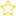See the live dashboard for CensusAtSchool 2023
(91586) NZC Level 8, NCEA Level 3: Statistics and Probability
External 4 credits
This standard is derived from Achievement Objective S8.4, Investigate situations that involve elements of chance.
• calculating and interpreting expected values and standard deviations of discrete random variables
• applying distributions such as the Poisson, binomial and normal

Methods are selected from those related to:

• discrete and continuous probability distributions
• mean and standard deviation of random variables
• distribution of true probabilities versus distribution of model estimates of probabilities versus distribution of experimental estimates of probabilities.

Students should be able to calculate the mean and standard deviation from a plot of the distribution of a discrete random variable, linking probabilities and areas under density functions for continuous outcomes.

• Keywords: Distributions, Probability, Probability distributions
• (Last updated: 15/05/18. Added: 15/05/18)
• ## Rate this resource:(No ratings yet)